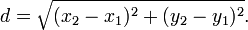×

# Distance Between Two Points Calculator

Enter two points (x1, y1) and (x2, y2):
 x1: y1: x2: y2:

## About Distance Between Two Points Calculator

The Distance Between Two Points Calculator is used to help you find the distance between two points.

## Distance Between Two Points Formula

The distance between any two points (x1, y1) and (x2, y2) is given by:## All of Our Miniwebtools (Sorted by Name):

•{{name}}
{{title}}

Miniwebtool

If you like Distance Between Two Points Calculator, please consider adding a link to this tool by copy/paste the following code:

Watch Videos: